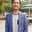Related Tags

javascript

# How to create a bubble chart using D3Shahpar Khan

D3 is an interactive JavaScript library for data visualization. It uses Scalar Vector Graphics (SVG) coupled with HTML and CSS to display charts and figures that illustrate the numeric data. You can also use D3 to make bubble charts. Here is a step-by-step guide on how to make a bubble chart using D3.

## Step 1: Dataset

Before even starting to code, we need a data set to base our chart on. For this example, we will take an array of objects where each object has five attributes: source, x, y, val, and color.

var data = [
{source:"Item 1", x: 100, y: 60, val: 1350, color: "#C9D6DF"},
{source:"Item 2", x: 30, y: 80, val: 2500, color: "#F7EECF"},
{source:"Item 3", x: 50, y: 40, val: 5700, color: "#E3E1B2"},
{source:"Item 4", x: 190, y: 100, val: 30000, color: "#F9CAC8"},
{source:"Item 5", x: 80, y: 170, val: 47500, color: "#D1C2E0"}
]


## Step 2: D3 and SVG container

We need to import the D3 script using the src attribute and then initialize our SVG container with the appropriate width and height.

<script src="https://d3js.org/d3.v4.min.js"></script>
<svg width="500" height="500"></svg>


## Step 3: Set dimensions

Make an svg variable and define its constraints keeping in mind the height and width we defined for our svg container in step 2.

    var svg = d3.select("svg")
.attr("width", 500)
.attr("height", 500);


## Step 4: Make bubbles

Now, we will make the bubbles. To do that, we first need to append circle to our svg and then give it the data to plot. Then, we need to specify the position for each bubble (cx and cy). After that, we need to specify the radius for each circle.

In a bubble chart, the circle area should be representative of its value, not its radius. Therefore, we first take the value’s square root and then divide it by pie ($\pi$) to get the corresponding radius. Finally, we color each circle according to the color specified in our data set.

    svg.selectAll("circle")
.data(data).enter()
.append("circle")
.attr("cx", function(d) {return d.x})
.attr("cy", function(d) {return d.y})
.attr("r", function(d) {
return Math.sqrt(d.val)/Math.PI
})
.attr("fill", function(d) {
return d.color;
});


Finally, we will add the labels to our bubble chart sections. But, first, we need to define the position where we need to write the labels, and for that, we will set the x and y coordinates accordingly. After that, we will append this text to our svg variable and style it accordingly.

    svg.selectAll("text")
.data(data).enter()
.append("text")
.attr("x", function(d) {return d.x+(Math.sqrt(d.val)/Math.PI)})
.attr("y", function(d) {return d.y+4})
.text(function(d) {return d.source})
.style("font-family", "arial")
.style("font-size", "12px")


RELATED TAGS

javascript

CONTRIBUTORShahpar Khan